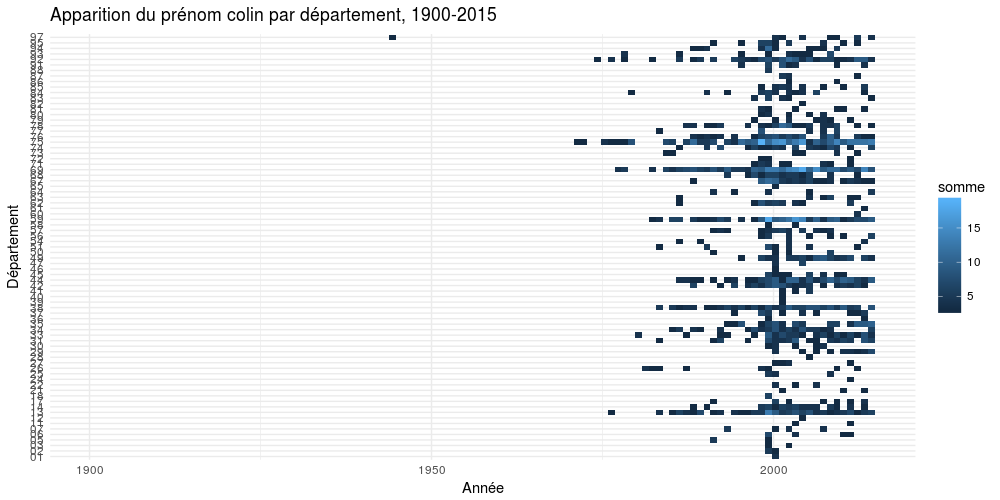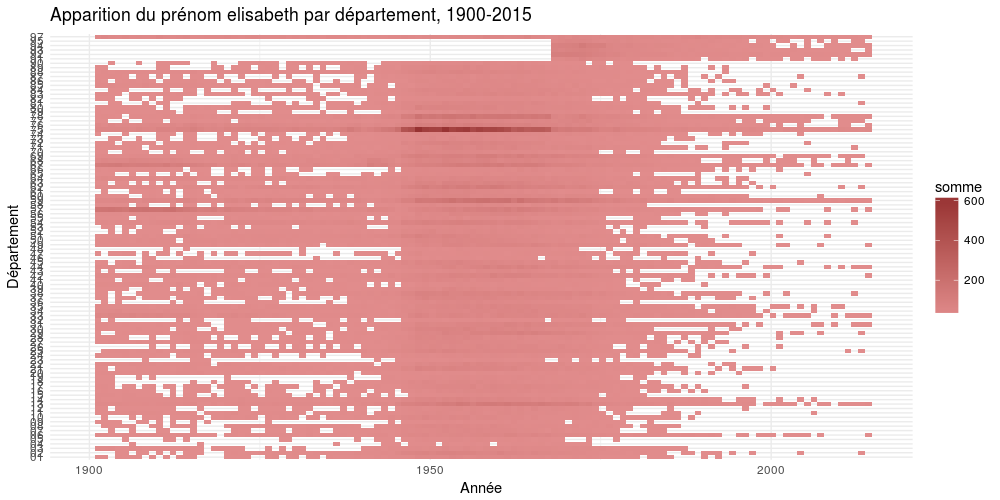# Create an heatmap with R and ggplot2

## Here a short tutorial for making a heatmap in R with ggplot2, inspired by several articles on databzh.

In this short post, I’ll show you how to create a heatmap with ggplot2 and R. We’ll visualise the evolution through time of a specific name in France. The dataset used in this article comes from data.gouv, and is unzipped outside R.

``````library(tidyverse)

name <- read.table("/home/colin/Téléchargements/dpt2015.txt", stringsAsFactors = FALSE, sep = "\t", encoding = "latin1", header = TRUE, col.names = c("sexe","prenom","annee","dpt","nombre")) %>%
na.omit()
name\$annee <- as.Date(name\$annee, "%Y")
``````

We now have a clean dataset of all the names in the several french departments, by year.

### Heatmap

A heatmap is created with the `geom_tile` geom from `ggplot`. Here how to create it step by step.

``````choix <- "COLIN"
name %>%
#Filter by name
filter(prenom == choix) %>%

#Group by two variables : year and dep
group_by(annee, dpt) %>%

#Summarise the sum of each name by year &amp; dep
summarise(somme = sum(nombre)) %>%

#Make sure you get rid of NA
na.omit() %>%

ggplot(aes(annee, dpt, fill = somme)) +

#HERE'S THE BIG GUY
geom_tile() +

scale_x_date(limits =  c(lubridate::ymd("1900-01-01"), lubridate::ymd("2015-01-01"))) +

#Here are some stuffs to make this plot pretty
xlab("Année") +
ylab("Département") +
labs(title = paste0("Apparition du prénom ", tolower(choix)," par département, 1900-2015")) +
theme_minimal()
``````So yeah, it’s that simple. Let’s try with another name.

(And of course, you can specify a different color scale for your plot)

``````choix <- "ELISABETH"
name %>%
filter(prenom == choix) %>%
group_by(annee, dpt) %>%
summarise(somme = sum(nombre)) %>%
na.omit() %>%
ggplot(aes(annee, dpt, fill = somme)) +
geom_tile() +
scale_x_date(limits =  c(lubridate::ymd("1900-01-01"), lubridate::ymd("2015-01-01"))) +
#Changer l'échelle de couleurs
scale_fill_gradient(low = "#E18C8C", high = "#973232") +
xlab("Année") +
ylab("Département") +
labs(title = paste0("Apparition du prénom ", tolower(choix)," par département, 1900-2015")) +
theme_minimal()

``````Pretty easy isn’t it?

Tags:

Categories:

Updated: